Mobile QR Code1. (Department of Electrical Engineering, Pohang University of Science and Technology (POSTECH), Pohang 37673, Korea)
2. (Memory Business, Samsung Electronics, Hwasung 18448, Korea)

Approximate computing, digital circuit, multiplier, Booth encoding, power/accuracy tradeoff

## I. INTRODUCTION

Approximate computing is a recently emerging discipline in which circuits are simplified or less important data bits or items are ignored in order to produce low power and/or high performance circuits (1) at the expense of a small loss in accuracy. In recent years, deep neural networks (DNNs) have been proposed that can perform highly accurate image identification and other artificial intelligence tasks. Such circuits use extremely large numbers of multiplications and are error-resilient (able to tolerate small errors in the subcircuits or data). For such error-resilient circuits, this paper examines an improved technique for fast and power-efficient approximate multiplication based on radix-4 Booth encoding.

Approximate multipliers can be implemented in various ways. Since the least significant bits of a number contribute less to the overall accuracy of a multiplication result, approximate circuits are typically used for several of the least significant bits. Liu ${et}$ ${al}$. (2) proposed approximate radix-4 Booth multiplier designs based on two simplified logic designs for producing partial product bits. During the partial product accumulation stage, approximate encoding methods were used for the least significant $\textit{p}$ bits, while exact encodings were used for the most significant 2$\textit{n}$${-}$$\textit{p}$ bits of the 2$\textit{n}$${-}bit product. Fixed width multipliers based on this idea have been proposed in (3)-(5). Jiang {et} {al}. (6) proposed two approximate radix-8 Booth designs. Leon {et} {al}. (7) proposed an approximate radix-4 design that applies approximations of very high radix (radix-64, radix-256, etc.) encodings for the least significant bits of a radix-4 multiplier and exact radix-4 encodings for the most significant bits. This paper proposes an approximate multiplier based on radix-4 Booth encoding that uses approximated \textit{multi-level} logic circuits (instead of 2-level circuits) in which intermediate terms are shared by many later terms. The proposed design results in better power-delay-product characteristics than previous designs while, at the same time, significantly improving the multiplication accuracy. By using an example image processing application (image multiplication), it is shown that the proposed multiplier is practical and produces satisfactory image outputs with high signal to noise ratios (PSNRs). ## II. PRELIMINARIES In signed binary multiplication, an \textit{n}$${-}$bit multiplier $B=b_{n-1}b_{n-2}\ldots b_{0}$ is multiplied with an $\textit{n}$${-}bit multiplicand A=a_{n-1}a_{n-2}\ldots a_{0} to produce a 2\textit{n}$${-}$bit product $P$. $\textit{P}$ is typically computed by adding partial products $P_{i}=2^{i}b_{i}A$ $\left(0\leq i\leq n-1\right).$ A combinational multiplier can be implemented by using an array or tree of up to 2$\textit{n}$${-}bit adders to add up to \textit{n} P_{i} terms formed by shifting (and possibly 2's complementing) sign{-}extended versions of the multiplicand. ### 1. Exact Multiplication using Booth Encoding Booth encoding (8) and its later forms, referred to as modified Booth or radix-\textit{r} Booth encoding, where \textit{r} is a power of 2, is a method of encoding the bits of a multiplier in a special nonbinary format of \textit{n} digits. These \textit{n} special multiplier digits can be used to form partial products in a manner that results in the need to use fewer addition or subtraction operations to form the same final product than with unencoded binary multiplication. Fewer adds and subtracts implies a shorter critical path delay and lower power usage. The effect of the reduction in add/subtracts is partially offset by the extra logic and delay required to produce the necessary radix-\textit{r} Booth encoded partial products, as shown in (9)-(13). By using radix-\textit{r} Booth encoding, the number of partial products that need to be added or subtracted can be reduced from \textit{n} to n/\log _{2} r. By using this simple logic, it would seem that it would be most beneficial to use the largest value of \textit{r} that can be accommodated. In fact, Jiang {et} {al}. has used this logic to argue for radix-\textit{r} Booth encoding with very large \textit{r} values (6). However, in general, because increasingly more complex logic is required to form the partial product terms with values of \textit{r} greater than 4, radix-4 Booth encoding is the most commonly used encoding method. In radix-4 Booth encoding, new partial product terms PP_{k} terms are formed based on the encoded forms of b_{2i} and b_{2i+1}, with k=i/2. Then the final product P is the sum of all PP_{k} terms, which are half as many as the original P_{i} terms. Previous researchers have proposed successively better methods, shown in Fig. 1 for \textit{n}=8 (selected to simplify the exposition), for re-encoding the partial products PP_{k} such that only about \textit{n}+2 bits need to be considered for each partial product. Fig. 1(a) shows the original set of n/2 PP_{k} partial product rows. Each PP_{k} is one of 0, P_{k}, 2P_{k}, -P_{k}, or -2P_{k}. For those partial product rows that require the use of -A, partial product bits \overline{a_{j}} (0\leq j\leq \mathrm{n}-1) are used and neg_{k}=1 is added on the “next” partial product row to achieve the same effect as a 2's complement operation. Blank spaces are 0 bits. {\blacksquare} is a partial product bit, which is always 0, a_{j}, or \overline{a_{j}} (0\leq j\leq n-1). The sign of PP_{k} is denoted as ▲ and is 0 or the msb of A or -A. Although Fig. 1 shows encodings for \textit{n} = 8-bit multiplication, the encodings for larger n values are essentially the same (the main differences are in the number of {\blacksquare} terms and partial product rows). Fig. 1. Encoding the partial products for efficient calculation: (a) the initial radix-4 Booth encoding design, (b) a design with fewer sign bits, (c) a design with simpler logic for the rightmost terms, and (d) a design with the last row removed. Blanks are 0's, ▲ is the sign bit, {\blacksquare} is a partial product term, and the rest are specially defined terms.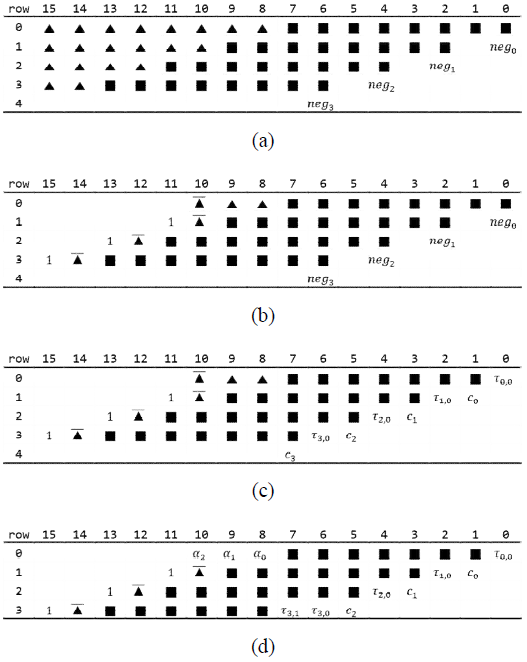The successive improvements adopted in Figs. 1(a)-(d) are as follows. In Fig. 1(b), the duplicated sign bits are eliminated by using the inverse of the sign bit and 1 (9). Fig. 1(c) shows how the neg_{k} entry can be simplified by using special c_{k} and \tau _{k,0} terms (10). Finally, Fig. 1(d) shows how the extra PP_{4} partial product row can be eliminated altogether by using special \tau _{\left\lceil \frac{n}{2}\right\rceil -1,1}, \alpha _{0}, \alpha _{1}, and \alpha _{2} terms. Kuang {et} {al}. (11) derived the logic equations and digital logic implementations for the \tau _{\left\lceil \frac{n}{2}\right\rceil -1,1}, \alpha _{0}, \alpha _{1}, and \alpha _{2} terms required. These terms are defined such that circuits based on Fig. 1(d) always produce the exact same results as Fig. 1(a). ### 2. Exact Partial Product Bit Equations In the following, “logical or" is represented by {\vee}, “logical and" is represented by {\wedge}, the exclusive-OR operation is denoted by {\oplus}, and the exclusive-NOR operation is denoted by {\odot}. In a partial product array, for each k-th row between 1 to {\lceil}$$\textit{n}$/2{\rceil}{-}1, the neg_{k}, one_{k}, and two_{k} common intermediate terms are defined as follows (11), (14). \begin{align*} \begin{array}{c} neg_{k}=b_{2k+1}\wedge \overline{\left(b_{2k}\wedge b_{2k-1}\right)}\\ \overline{one_{k}}=b_{2k}\odot b_{2k-1}\\ \overline{two_{k}}=\overline{\left(\overline{b_{2k+1}}\wedge b_{2k}\wedge b_{2k-1}\right)\vee \left(b_{2k+1}\wedge \overline{b_{2k}}\wedge \overline{b_{2k-1}}\right)}\\ na_{k,j}=a_{j}\odot b_{2k+1}\\ \overline{\epsilon }=\begin{cases} \\ \end{cases} \begin{array}{cc} a_{1} & \mathrm{if} \left(\overline{a_{0}\wedge b_{n-1}}==0\right)\\ \overline{a_{1}} & \text{otherwise} \end{array}\\ d_{\left\lceil \frac{n}{2}\right\rceil -1}=\left(\overline{\overline{b_{n-1}}\vee a_{0}}\right)\wedge \overline{\left(b_{n-1}\vee a_{1}\right)\wedge \left(b_{n-2}\vee a_{1}\right)\wedge \left(b_{n-2}\vee b_{n-3}\right)}\end{array} \end{align*} Using the intermediate terms defined above, the following logic equations can be derived for the exact forms of the partial product bits p_{k,j} ({\blacksquare}), sign bits s_{k} (▲), and special bits. \begin{align*} \begin{array}{c} p_{k,j}^{\left(\textit{exact}\right)}=\overline{\left(na_{k,j-1}\vee \overline{two_{k}}\right)\wedge \left(na_{k,j}\vee \overline{one_{k}}\right)}\\ s_{k}^{\left(\textit{exact}\right)}=\overline{\left(na_{k,n-1}\vee \overline{two_{k}}\right)\wedge \left(na_{k,n-1}\vee \overline{one_{k}}\right)}\\ c_{k}^{\left(\textit{exact}\right)}=\overline{\overline{neg_{k}}\vee \left(one_{k}\wedge a_{0}\right)}\\ \tau _{k,0}^{\left(\textit{exact}\right)}=\overline{\overline{one_{k}}\vee \overline{a_{0}}}\\ \tau _{\left\lceil \frac{n}{2}\right\rceil -1,1}^{\left(\textit{exact}\right)}=\overline{\left(\overline{one_{\left\lceil \frac{n}{2}\right\rceil -1}}\vee \overline{\epsilon }\right)\wedge \left(\overline{two_{\left\lceil \frac{n}{2}\right\rceil -1}}\vee \overline{a_{0}}\right)}\\ \alpha _{2}^{\left(\textit{exact}\right)}=\overline{s_{0}\wedge \overline{d_{\left\lceil \frac{n}{2}\right\rceil -1}}}\\ \alpha _{1}^{\left(\textit{exact}\right)}=\overline{\alpha _{2}^{\left(\textit{exact}\right)}}\\ \alpha _{0}^{\left(\textit{exact}\right)}=s_{0}\odot \overline{d_{\left\lceil \frac{n}{2}\right\rceil -1}} \end{array} \end{align*} ### 3. Approximate Radix-4 Booth Multiplication An approximate radix-4 Booth multiplier can be designed by using approximate Booth encoding starting from one of the Booth encodings shown in Fig. 1. The method proposed by Liu {et} {al}. (2) uses approximate designs for the terms in the Booth encoding shown in Fig. 1(b), while the method proposed in this paper uses approximate designs for the terms in the Booth encoding shown in Fig. 1(d). The determination of which is the best method is ultimately determined by the power, delay, and accuracy characteristics of the resulting circuit. Another useful technique used for approximate radix-4 Booth multiplication is the use of approximation and accurate regions, with these regions defined by partitioning the columns of the partial product addition array. Thus, the columns of the partial product addition array can be partitioned into two regions starting from the rightmost bits: a truncated region and an exact (circuit) region (3), (5) or an approximate region and an exact region (2). The reason the focus is placed on partitioning based on columns is that the rightmost columns correspond to the least significant bits and the leftmost columns correspond to the most significant bits of the final product. Thus, if a final product bit is incorrect, it will only change the result by a small amount (from the correct result) if the incorrect product bit is one of the rightmost bits in the final product. Higher power savings can be achieved (at the cost of higher error levels) by using more bits in the truncated and approximate regions and by using more aggressive approximation circuits. Thus, in the proposed design, as shown in Fig. 2, the partial product array is partitioned into three parts from the leftmost to the rightmost bits: accurate, approximated, and truncated. ## III. PROPOSED DESIGN ### 1. Approximated Partial Product Bits The complete proposed approximate radix-4 Booth multiplier design is shown in Fig. 2. Although an 8-bit multiplier is shown, n-bit multipliers with larger n values will follow the same patterns. This multiplier uses the knowledge accumulated from previous designs in order to arrive at a design suitable for error-resilient applications such as those using DNNs. The \textit{novel} aspect of this approach is the introduction of new approximation circuitry that use \textit{multi-level} logic and \textit{shared intermediate terms} in order to produce designs with better power/accuracy trade-offs than the previous best designs. The proposed multiplier consists of three stages. In Stage 1, the partial product bits are partitioned into three regions: exact, approximate, and truncated. The \textit{new} approximate logic circuits proposed in this paper are used in the approximate region of Stage 1. The cut-off lines for the exact, approximate, and truncated regions can be adjusted to produce multipliers with varying degrees of accuracy and power usage. In Stage 2, 4-2 compressors (15) are used in one or more phases to reduce the partial products to two final partial products (2). In Stage 3, a 2-1 compressor, such as a hierarchical carry lookahead adder, is used to produce the final 2\textit{n}{-}bit product (2).

Unlike Liu ${et}$ ${al}$.'s method (2), which bases its approximate circuits on the partial product terms shown in Fig. 1(b), this paper proposes new approximate circuits based on the partial product terms shown in Fig. 1(d), which is the encoding proposed by Kuang ${et}$ ${al}$. (11). Another major difference in the proposed approach is that new approximate circuits are proposed for the $p_{k,j}$ partial product bits (denoted by ${\blacksquare}$ in Fig. 1) and the “special” terms $\tau _{\left\lceil \frac{n}{2}\right\rceil -1,1}$$\textit{,} \alpha _{0}$$\textit{,}$ $\alpha _{1}$$\textit{,} and \alpha _{2} in Fig. 1(d). Since these latter special terms all require fairly “heavy” digital logic implementations for exact calculations, using simplified circuits for such special terms has a significant effect on the overall circuit complexity. In general, a partial product term in the set of partial product rows produced by a radix-4 Booth encoding method is dependent on six variables: b_{2k+1}$$\textit{,}$ $b_{2k}$$\textit{,} b_{2k-1}$$\textit{,}$ $a_{j}$$\textit{,} a_{j-1}$$\textit{,}$ and $a_{n-1}$.

Since a 2-level AND-OR circuit with six variables can be quite “heavy,” Huang ${et}$ ${al}$. (14) proposed a sequence of equations that can be used to produce multi-level circuit implementations of the required partial product terms that has the additional advantage of reusing intermediate terms for all elements in a partial product row. This paper proposes new approximate circuits based on modifications of these multi-level circuits with shared intermediate terms.

### 2. Efficient Approximations for Partial Product Bits Terms

When logic circuits based on the exact equations shown in the previous subsection are used, the resulting circuits are particularly complex (with longer resulting delays and increased power consumption) for the $\tau _{\left\lceil \frac{n}{2}\right\rceil -1,1}^{(\textit{exact})}$$\textit{,} \alpha _{0}^{(\textit{exact})}$$\textit{,}$ $\alpha _{1}^{(\textit{exact})}$ and $\alpha _{2}^{(\textit{exact})}$ terms. Also, the $p_{k,j}^{(\textit{exact})}$ terms constitute the most heavily used terms in the partial product array of Fig. 1(d), upon which the proposed design is based. Thus, efficient approximate circuits are proposed for these five types of partial product terms.

Table 1. Truth table for efficient approximation of $\tau _{\left\lceil \frac{n}{2}\right\rceil -1,1}$

 $b_{2k+1}b_{2k}b_{2k-1}$ $\tau _{\left\lceil \frac{n}{2}\right\rceil -1,1}^{\left(\textit{exact}\right)}$ $\tau _{\left\lceil \frac{n}{2}\right\rceil -1,1}^{\left(\textit{approx}\right)}$ 000 0 0 001 $a_{1}$ $a_{1}$ 010 $a_{1}$ $a_{1}$ 011 $a_{0}$ $a_{0}$ 100 $a_{0}$ $a_{0}$ 101 $a_{1}\oplus a_{0}$ $a_{1}$ 110 $a_{1}\oplus a_{0}$ $a_{1}$ 111 0 0

Fig. 3. Proposed $\tau _{\left\lceil \frac{n}{2}\right\rceil -1,1}^{(\textit{approx})}$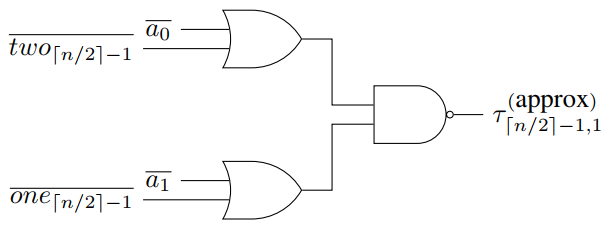Targeting the $\tau _{\left\lceil \frac{n}{2}\right\rceil -1,1}^{(\textit{exact})}$ term first, the complexity of the logic for this term derives mainly from the use of $\overline{\epsilon }$, which is used for only $\tau _{\left\lceil \frac{n}{2}\right\rceil -1,1}^{(\textit{exact})}$. However, by referring to Table 1 and Fig. 3, it can be seen that $\tau _{\left\lceil \frac{n}{2}\right\rceil -1,1}^{(\textit{exact})}$can be approximated without using $\overline{\epsilon }$ with changes to only 2 (out of 8) truth table entries.

Next, for the $\alpha _{0}, \alpha _{1},$ and $\alpha _{2}$ terms, efficient approximations can be derived by using the K-maps shown in Tables 2-4 and Fig. 4. The complexity of these terms derive mainly from the use of $d_{\left\lceil \frac{n}{2}\right\rceil -1}$. By using the K-maps shown, it can be seen that $d_{\left\lceil \frac{n}{2}\right\rceil -1}$can be approximated by the much simpler expression $d^{(\textit{approx})}$ = $\mathrm{a}_{1}\vee \mathrm{a}_{0}\vee \overline{\mathrm{b}_{\mathrm{n}-1}}$.

Table 2. Truth table for efficient approximation of $\alpha _{0}$

 $s_{0}a_{1}a_{0}$ $b_{n-1}b_{n-2}b_{n-3}$ 000 001 011 010 110 111 101 100 000 0 0 0 0 1 ① 1 1 001 0 0 0 0 0 0 0 0 010 0 0 0 0 0 0 0 0 011 0 0 0 0 0 0 0 ⓪ 110 1 1 1 1 1 1 1 ① 111 1 1 1 1 1 1 1 1 101 1 1 1 1 1 1 1 1 100 1 1 1 1 0 ⓪ 0 0

Table 3. Truth table for efficient approximation of $\alpha _{1}$

 $s_{0}a_{1}a_{0}$ $b_{n-1}b_{n-2}b_{n-3}$ 000 001 011 010 110 111 101 100 000 0 0 0 0 0 0 0 0 001 0 0 0 0 0 0 0 0 010 0 0 0 0 0 0 0 0 011 0 0 0 0 0 0 0 0 110 1 1 1 1 1 1 1 ① 111 1 1 1 1 1 1 1 1 101 1 1 1 1 1 1 1 1 100 1 1 1 1 0 ⓪ 0 0

Table 4. Truth table for efficient approximation of $\alpha _{2}$

 $s_{0}a_{1}a_{0}$ $b_{n-1}b_{n-2}b_{n-3}$ 000 001 011 010 110 111 101 100 000 1 1 1 1 1 1 1 1 001 1 1 1 1 1 1 1 1 010 1 1 1 1 1 1 1 1 011 1 1 1 1 1 1 1 1 110 0 0 0 0 0 0 0 ⓪ 111 0 0 0 0 0 0 0 0 101 0 0 0 0 0 0 0 0 100 0 0 0 0 1 ① 1 1

Fig. 4. Proposed $\alpha _{0}^{(\textit{approx})}$, $\alpha _{1}^{(\textit{approx})}$, and $\alpha _{2}^{(\textit{approx})}$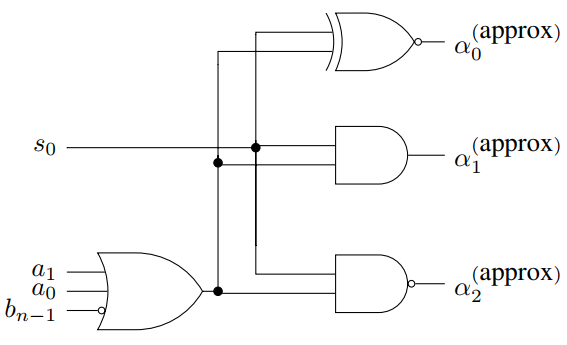Table 5. Truth table for efficient approximation of $p_{k,j}^{(\textit{exact})}$

 $b_{2k+1}b_{2k}b_{2k-1}$ $p_{k,j}^{\left(\textit{exact}\right)}$ $p_{k,j}^{\left(1\right)}$ $p_{k,j}^{\left(2\right)}$ $p_{k,j}^{\left(3\right)}$ $p_{k,j}^{\left(4\right)}$ $p_{k,j}^{\left(5\right)}$ $p_{k,j}^{\left(6\right)}$ 000 0 0 $a_{j-1}$ 0 $a_{j}$ 0 0 001 $a_{j}$ $a_{j}$ $a_{j}\vee a_{j-1}$ $a_{j}$ $a_{j}$ 1 0 010 $a_{j}$ $a_{j}$ $a_{j}\vee a_{j-1}$ $a_{j}$ $a_{j}$ 1 0 011 $a_{j-1}$ 1 $a_{j-1}$ 0 $a_{j-1}$ $a_{j-1}$ $a_{j-1}$ 100 $a_{j-1}$ 1 $a_{j-1}$ 0 $a_{j-1}$ $a_{j-1}$ $a_{j-1}$ 101 $\overline{a_{j}}$ $\overline{a_{j}}$ $\overline{a_{j}\vee a_{j-1}}$ $\overline{a_{j}}$ $\overline{a_{j}}$ 1 0 110 $\overline{a_{j}}$ $\overline{a_{j}}$ $\overline{a_{j}\vee a_{j-1}}$ $\overline{a_{j}}$ $\overline{a_{j}}$ 1 0 111 0 0 $\overline{a_{j-1}}$ 0 $\overline{a_{j}}$ 0 0

Finally, the all-important $p_{k,j}^{\left(\textit{exact}\right)}$term can be approximated by using the truth table of Table 5. This table shows various possible ways (with equations shown below) for approximating $p_{k,j}^{\left(\textit{exact}\right)}$ without incurring too much error.

\begin{align*} \begin{array}{rr} p_{k,j}^{\left(1\right)} & =\left(two_{k}\right)\vee \left(one_{k}\wedge \overline{na_{k,j}}\right)\\ p_{k,j}^{\left(2\right)} & =\left(\overline{na_{k,j-1}}\right)\vee \left(one_{k}\wedge \overline{na_{k,j}}\right)\\ p_{k,j}^{\left(3\right)} & =\left(one_{k}\wedge \overline{na_{k,j}}\right)\\ p_{k,j}^{\left(4\right)} & =\left(two_{k}\wedge \overline{na_{k,j-1}}\right)\vee \left(\overline{na_{k,j}}\right)\\ p_{k,j}^{\left(5\right)} & =\left(two_{k}\wedge \overline{na_{k,j-1}}\right)\vee \left(one_{k}\right)\\ p_{k,j}^{\left(6\right)} & =\left(two_{k}\wedge \overline{na_{k,j-1}}\right) \end{array} \end{align*}

Referring to the equations shown above and Table 5, it can be seen that these approximations result in 2 or 4 different entries (out of 8 entries) in this truth table. Of the approximations that result in 2 different entries, $p_{k,j}^{\left(3\right)}$ results in the simplest logic circuit. Thus, the approximate circuit based on $p_{k,j}^{\left(3\right)}$ , shown in Fig. 5, is used.

To summarize, the simplified logic equations for the approximations used in the proposed circuit are as shown below.

\begin{align*} \begin{array}{rr} \tau _{\left\lceil \frac{n}{2}\right\rceil -1,1}^{\left(\textit{approx}\right)} & =\overline{\left(\overline{one_{\left\lceil \frac{n}{2}\right\rceil -1}}\vee \overline{a_{1}}\right)\wedge \left(\overline{two_{\left\lceil \frac{n}{2}\right\rceil -1}}\vee \overline{a_{0}}\right)}\\ d^{\left(\textit{approx}\right)} & =a_{1}\vee a_{0}\vee \overline{b_{n-1}}\\ \alpha _{2}^{\left(\textit{approx}\right)} & =\overline{s_{0}\wedge d^{\left(\textit{approx}\right)}}\\ \alpha _{1}^{\left(\textit{approx}\right)} & =s_{0}\wedge d^{\left(\textit{approx}\right)}\\ \alpha _{0}^{\left(\textit{approx}\right)} & =s_{0}\odot d^{\left(\textit{approx}\right)}\\ p_{k,j}^{\left(\textit{approx}\right)} & =\overline{na_{k,j}\vee \overline{one_{k}}}\\ s_{k}^{\left(\textit{approx}\right)} & =p_{k,n-1}^{\left(\textit{approx}\right)} \end{array} \end{align*}

The proposed multiplier design was compared to the most recent comparable approximate radix-4 Booth approximate multiplier designs: the two designs proposed by Liu ${et}$ ${al}$. (2) and the high-radix approximate design proposed by Leon ${et}$ ${al}$. (7). The reason that only these previous designs were compared to the proposed design is because Leon ${et}$ ${al}$. (7) and Liu ${et}$ ${al}$. (2) have already shown that their designs were better than all previously proposed approximate radix-4 Booth multiplier designs.

The four approximate radix-4 Booth multiplier designs compared are labeled R4ABM1, R4ABM2, RAD, and R4IABM (proposed). The two designs proposed by Liu ${et}$ ${al}$., R4ABM1 and R4ABM2, offer a trade-off between power and accuracy. To implement a single partial product bit $p_{k,j}$, R4ABM2 uses one exclusive-OR gate while R4ABM1 uses the AND of the output of two exclusive-OR gates. Thus, R4ABM2 offers lower power usage at the expense of a decrease in output accuracy as opposed to R4ABM1. The design proposed by Leon ${et}$ ${al}$. , RAD, uses approximate radix-$2^{l}$ encoding for the least significant $l$ bits and exact radix-4 encoding for the most significant $\textit{n}$ ${-}$$l bits of the multiplier. Finally, the R4IABM (proposed) design incorporates all of the approximate partial bit designs proposed in this paper. ## VI. EVALUATION The proposed multiplier design can be configured based on the sizes of the truncated and approximate regions used. Two parameters, q_{1} and q_{2}, are used, where output bits q_{2}-1 through 0 are in the truncated region, q_{1}-1 through q_{2} are in the approximate region, and 2\textit{n}$$-1$ through $q_{1}$ are in the exact region. For example, if $q_{1}$ is 0, then all columns are in the exact region. On the other hand, if $q_{1}$ is 2$\textit{n}$, then there is no exact region. Multiplier designs were evaluated for all possible combinations of $q_{1}$ and $q_{2}$ for $\textit{n}$=8 and $\textit{n}$=16 bit multipliers. A representative sample of the results for these various design combinations are shown in this section.

Fig. 6. Various possible approximate 8-bit multiplier designs (the dashed line in (b) shows an example practical design point, which also corresponds to the design point with maximum improvement of the 4AIABM method over the RAD method).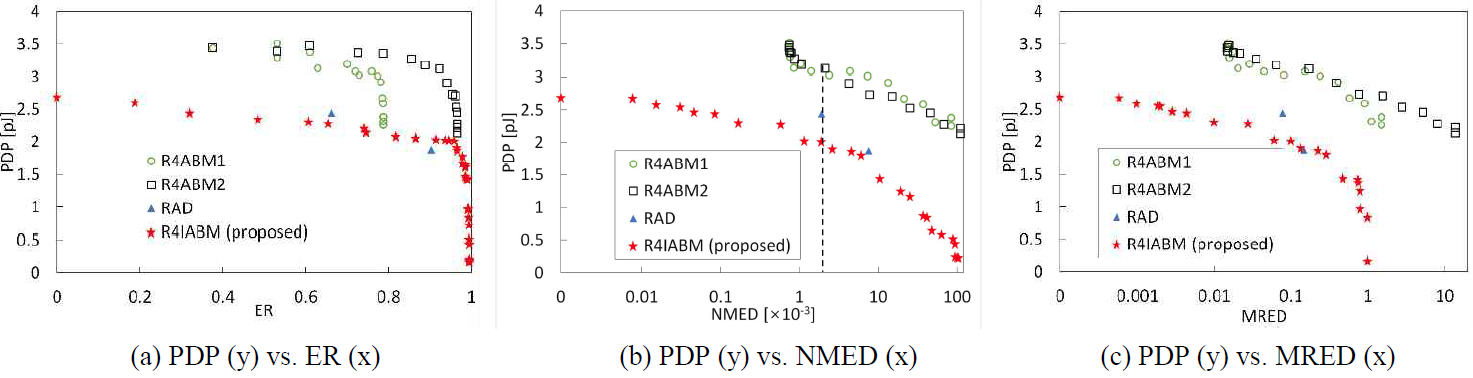Fig. 7. Various possible approximate 16-bit multiplier designs (the dashed line in (b) shows an example practical design point, which also corresponds to the design point with maximum improvement of the 4AIABM method over the RAD method).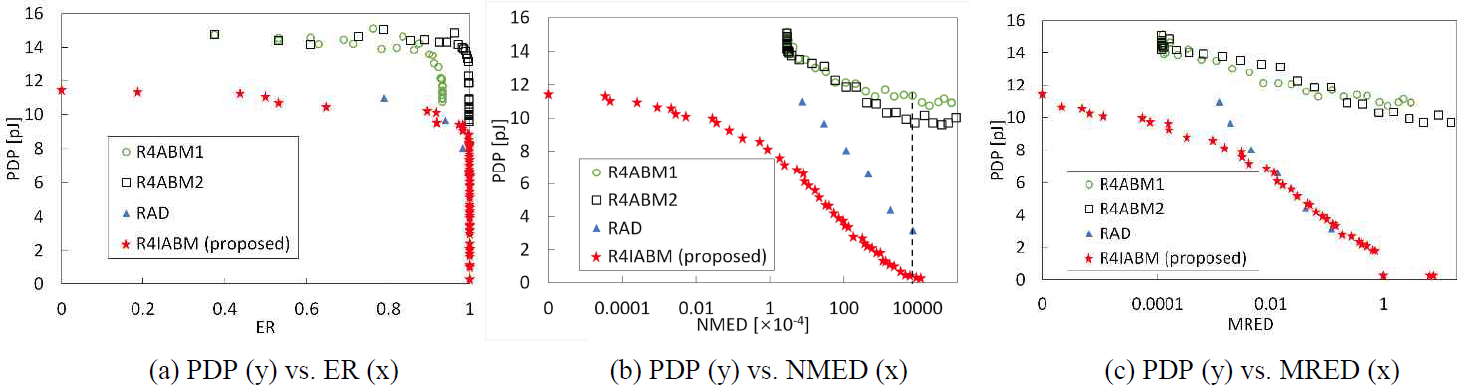### 1. Evaluation Metrics

The metrics used to evaluate the multiplier designs under comparison are the power required, the worst-case multiplication delay, circuit area and the degree of error introduced by the approximation circuits used. In order to estimate power and delay, the designs being compared have all been implemented at the logic gate level using Verilog HDL, and synthesized with Synopsys Design Compiler using a CMOS 65nm cell library, 1.25V supply voltage, and room temperature operating conditions. All possible input parameter combinations were evaluated.

Traditionally, the quality of a digital circuit design targeted for ASIC implementation is evaluated by measuring the chip area, power, and critical path delay. An overall evaluation design quality measure that is typically used is the ${power}$ ${delay}$ ${product}$ ${(PDP)}$, which is the product of the power required with the critical path delay.

\begin{equation*} PDP=\textit{Power}\times \textit{Delay} \end{equation*}

For an approximate digital logic circuit design, the accuracy of the output produced by the approximate circuit, when compared to the output of an exact circuit, is clearly of importance. For an approximate multiplier, accuracy can be measured with respect to the ${error}$ ${rate}$ ${(ER)}$, the ${normalized}$ ${mean}$ ${error}$ ${distance}$ ${(NMED)}$, and the ${mean}$ ${relative}$ ${error}$ ${distance}$ ${(MRED)}$. ER is the ratio of possible input vectors that result in incorrect outputs. NMED is the mean absolute difference between correct and approximate results (error distance) normalized by the maximum output of the exact design. MRED is the average of the error distances divided by the absolute exact output values.

Table 6. Area comparison of 8-bit designs

 Previous design Area ($\mu m^{2}$) Proposed design (R4IABM) Area ($\mu m^{2}$) Type p $q_{1}$ $q_{2}$ R4ABM1 4 1308.48 4 0 848.32 8 1454.40 8 0 878.72 12 1045.76 8 4 812.16 R4ABM2 4 1522.88 8 8 475.20 8 1321.60 12 0 824.96 12 1005.12 12 4 752.96 RAD16 958.40 12 8 393.28 RAD64 792.00 12 12 137.92

Table 7. Area comparison of 16-bit designs

 Previous design Area ($\mu m^{2}$) Proposed design (R4IABM) Area ($\mu m^{2}$) Type p $q_{1}$ $q_{2}$ R4ABM1 4 5017.60 4 0 3436.80 8 4977.60 8 0 3423.04 16 4718.40 8 4 2844.48 24 4223.36 8 8 3139.52 R4ABM2 4 5556.16 16 0 3344.32 8 5064.00 16 8 3340.80 16 4752.64 16 16 1768.64 24 3308.48 24 0 3072.32 RAD16 3348.80 24 8 2652.80 RAD64 2962.56 24 16 1586.88 RAD256 2528.96 24 24 469.12 RAD1024 2347.52 32 0 2974.40 RAD4096 1694.72 32 8 2929.28 RAD16384 1338.56 32 16 1532.80

### 2. Evaluation Results

The accuracy and resource usage data for 8/16-bit multipliers designed using (2), (7) and the proposed method have been evaluated in Figs.~6 and 7 and Tables 6 and 7. These results are shown with various values for the approximation factor $\textit{p}$ and $l$ for the previous designs and the region separation factors $q_{1}$ and $q_{2}$ (note that $p=q_{1}$) for the proposed designs. Since there are 153 (561) possible combinations of $q_{1}$ and $q_{2}$ values for 8-bit (16-bit) multipliers, only a representative subset of these combinations are shown in the figures and tables. However, in our experiments, all 153 (561) multipliers have been implemented at the logic gate level and synthesized one by one.

### 3. Comparison Summaries

From the figures shown, several observations can be made regarding the various approximate multipliers that have been compared. Except for a very few versions of the R4IABM (proposed) designs, ${all}$ of the approximate radix-4 Booth multipliers have extremely high error rates ER, ranging from about 40% to almost 100%. However, by referring to the NMED plots, it can be seen that the average error ${distance}$ (difference from the correct products) is fairly small (NMED values of less than about 0.1) for most of the designs shown. This shows that approximate radix-4 Booth multipliers tend to produce a high ratio of incorrect product results, but the ${magnitude}$ of those errors can be quite small on average.

Overall, the proposed approximate radix-4 Booth multiplier design shows clear improvements over other recently proposed alternative designs. For a fixed level of accuracy (modeled by NMED), the power and delay characteristics (modeled by PDP) show improvements of up to 30.3% (96.4%) over the previous 8-bit (16-bit) designs proposed by Liu et al. and up to 13.4% (88.9%) over Leon et al.’s 8-bit (16-bit) designs. These design points are on the dashed lines shown in Figs.~6(b) and ~7(b).

Tables 6 and 7 show that, given the same number of columns for the exact and approximate regions, R4IABM occupies a smaller area than the previous methods in almost all cases. The R4IABM methods result in lower area than the previous R4ABM methods in all cases. There are a few cases when the ${RAD}$ $2^{\kappa }$ methods result in lower area than the R4IABM methods. According to (7), ${RAD}$ $2^{\kappa }$ ${reduces}$ $\frac{\kappa }{2}-1$ partial product rows. The ${RAD}$ $2^{\kappa }$ methods reduce rows while the proposed R4IABM method reduces columns. Thus, although the area requirements of the two types of methods are not directly comparable, it can nevertheless be seen that the ${RAD}$ $2^{\kappa }$ methods can sometimes produce lower area overhead designs than R4IABM. However, the R4IABM still has an advantage over the ${RAD}$ $2^{\kappa }$ methods in terms of power, delay, and accuracy as shown in Figs. 6 and 7.

Fig. 8. Image multiplication using the 8-bit R4IABM (proposed) design.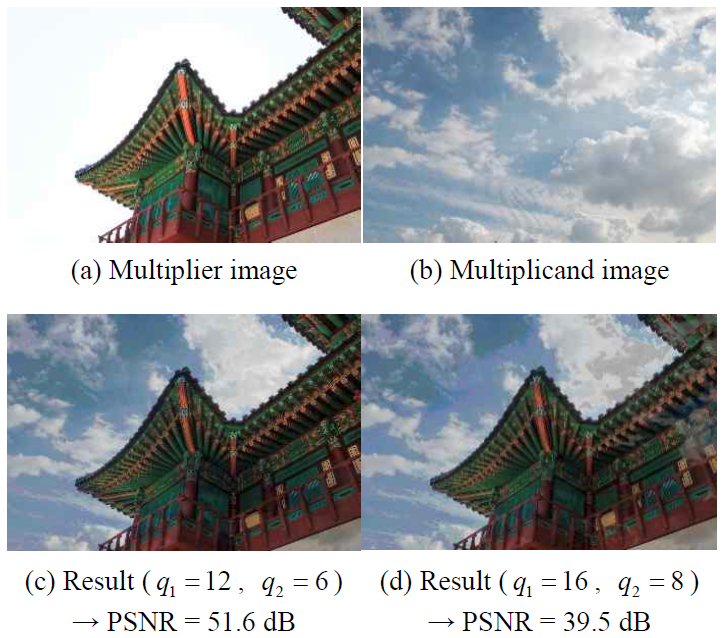### 4. An Image Multiplication Example

Given the levels of ER, NMED, and MRED achieved by the proposed method, it is reasonable to question whether approximate multiplier designs based on the proposed method ${can}$ be used in actual applications (16). In order to answer this question, the authors have implemented the proposed method in software and used it to test an example image processing application.

In the target image processing application, the two images shown in Figs. 8(a) and (b) were multiplied together in a pixel by pixel manner to produce a combined image. The result figures and ${Peak}$ ${Signal-to-Noise}$ ${Ratio}$ ${(PSNR)}$ are shown in Figs.~8(c) and (d) for approximate radix-4 multiplication (only two $q_{1}$ and $q_{2}$ combinations are shown here for brevity). Upon close inspection, it was observed that for combinations less than about ($q_{1}=12$, $q_{2}=6$), there was hardly any distortion, while slight distortions were detectable for larger $q_{1}$ and $q_{2}$ values, as evidenced by the multiplication result with ($q_{1}=16$, $q_{2}=8$), which resulted in PSNR = 39.5~dB. These results validate the practicality of the proposed approach.

## V. CONCLUSION

This paper proposes a new approximate multiplier design that has significantly improved power-accuracy trade-off characteristics when compared to previously proposed designs.

Accuracy and hardware usage characteristics are measured using the Synopsys Design Compiler tool with a CMOS 65nm cell library. When compared to the previous best approximate multiplier designs, the proposed 8-bit (16-bit) design improves the power delay product by 13.4% to 30.3% (88.9% to 96.4%) given similar accuracy levels, as measured by the normalized mean error distance. In addition, the proposed method offers a wide range of options for trading off power usage levels for accuracy. Finally, the proposed method is shown to produce acceptable accuracy results for practical applications such as image multiplication.

### ACKNOWLEDGMENTS

This work was supported by Samsung Electronics and the Samsung Research Funding & Incubation Center of Samsung Electronics under Project Number SRFC-TB1703-07.

### REFERENCES

1
Xu Q., Mytkowicz T., Kim N. S., 2016, Approximate computing: A survey, IEEE Design & Test, Vol. 33, No. 1, pp. 8-222
Liu W., Qian L., Wang C., Jiang H., Han J., Lombardi F., 2017, Design of approximate radix-4 booth multipliers for error-tolerant computing, IEEE Transactions on Computers, Vol. 66, No. 8, pp. 1435-14413
Zhang Z., He Y., 2017, A low error energy-efficient fixed-width booth multiplier with sign−digit-based conditional probability estimation, IEEE Transactions on Circuits and Systems II4
Li C.-Y., Chen Y.-H., Chang T.-Y., Chen J.-N., Express Briefs, A probabilistic estimation bias circuit for fixed-width booth multiplier and its dct applications, IEEE Transactions on Circuits and Systems II, Vol. 58, No. 4, pp. 215-2195
Juang T.-B., Hsiao S.-F., Express Briefs, Low-error carry-free fixed-width multipliers with low-cost compensation circuits, IEEE Transactions on Circuits and Systems II, Vol. 52, No. 6, pp. 299-3036
Jiang H., Han J., Qiao F., Lombardi F., 2016, Approximate radix-8 booth multipliers for low-power and high-performance operation, IEEE Transactions on Computers, Vol. 65, No. 8, pp. 2638-6447
Leon V., Zervakis G., Soudris D., Pekmestzi K., 2018, Approximate hybrid high radix encoding for energy-efficient inexact multipliers, IEEE Transactions on Very Large Scale Integration (VLSI) Systems, Vol. 26, No. 3, pp. 421-4308
Booth A. D., 1951, A signed binary multiplication technique, The Quarterly Journal of Mechanics & Applied Mathematics, Vol. 4, No. 2, pp. 236-2409
Fadavi-Ardekani J., 1993, M*N booth encoded multiplier generator using optimized wallace trees, IEEE Transactions on Very Large Scale Integration (VLSI) Systems, Vol. 1, No. 2, pp. 120-12510
Yeh W.-C., Jen C.-W., 2000, High-speed booth encoded parallel multiplier design, IEEE Transactions on Computers, Vol. 49, No. 7, pp. 692-70111
Kuang S.-R., Wang J.-P., Guo C.-Y., 2005, Modified booth multipliers with a regular partial product array, IEEE Transactions on Circuits and Systems II, Express Briefs, Vol. 56, No. 5, pp. 404-40812
Kang J.-Y., Gaudiot J.-L., 2006, A simple high-speed multiplier design, IEEE Transactions on Computers, Vol. 55, No. 10, pp. 1253-125813
Patil P. K., Kumre L., 2013, High speed-low power radix-8 booth decoded multiplier, International Journal of Computer Applications, Vol. 73, No. 1414
Huang Z., Ercegovac M. D., 2005, High-performance low-power left-to-right array multiplier design, IEEE Transactions on Computers, Vol. 54, No. 3, pp. 272-28315
Ha M., Lee S., 2018, Multipliers with approximate 4-2 compressors and error recovery modules, IEEE Embedded Systems Letters, Vol. 10, No. 1, pp. 6-916
Rhee S.-H., Lee H.-C., Kim S.-H., Choi B.-T., Lee S.-S., Choi S.-J., 2005, A System-on-a-Chip Design for Digital TV, Journal of Semiconductor Technology and Science, Vol. 5, No. 4, pp. 249-254## Author

Kim received B.S. and M.S. degrees in electrical engineering from Pohang University of Science and Technology (POSTECH), Pohang, South Korea, in 2014 and 2016, respectively.

He is currently pursuing a Ph.D. degree in electrical engineering at POSTECH, Pohang, Korea. His current research interests include algorithms and architectures for embedded systems with FPGA, SoC based WSN, and NoC.

received a B.S. degree in electronic materials engineering from Kwangwoon University, Seoul, Korea, in 2016.

He is currently pursuing an M.S degree in electrical engineering at POSTECH, Pohang, Korea. His current research interests include algorithms and architectures for digital systems.

(M'14) received the B.S., M.S., and Ph.D. degrees in electrical engineering from Korea Advanced Institute of Science and Technology (KAIST), Daejeon, Korea, in 2008, 2010, and 2014, respectively.

Since 2017, he has been an assistant professor in the department of Electrical Engineering, Pohang University of Science and Technology (POSTECH), Pohang, Korea.

Prior to joining POSTECH, he was with Interuniversity Microelectronic Center (IMEC), Leuven, Belgium, from 2014 to 2015, where he researched reconfigurable SoC platforms for software-defined radio systems.

From 2015 to 2016, he was with the faculty of the department of Electronic Engineering, Kwangwoon University, Seoul, Korea. His current research interests include the algorithms and architectures for embedded processors, intelligent mobile systems, advanced error-correction codes, and mixed-signal integrated circuits.

(M'88) received the B.S.E.E. degree with highest distinction from the University of Kansas, Lawrence, in 1985 and the M.S.E. and Ph.D. degrees from the University of Michigan, Ann Arbor, in 1987 and 1990, respectively.

He is currently a Professor in the Department of Electrical Engineering at the Pohang University of Science and Technology (POSTECH), Pohang, South Korea.

Prior to this appointment, he was an Assistant Professor in the Department of Electrical Engineering at the University of Delaware in Newark, Delaware, U.S.A. He has been a Visiting Scientist at the IBM T. J. Watson Research Center, a visiting researcher at the DREAM Laboratory in the University of California at Irvine, and a visiting researcher at Samsung Electronics in Suwon, Korea.

His current research interests are in hardware implementations of deep neural networks, approximate computing, and fault-tolerant system design.